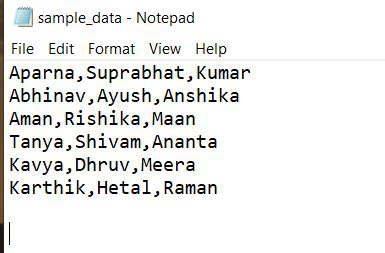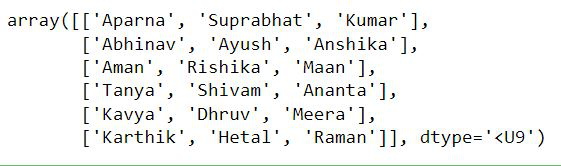# How to Read CSV Files with NumPy?

• Last Updated : 19 Dec, 2021

Dataset in use:## Method 1: Using loadtxt method

To import data from a text file, we will use the NumPy loadtxt() method. To use this function we need to make sure that the count of entries in each line of the text document should be equal. In Python, numpy.load() is used to load data from a text file, with the goal of being a quick read for basic text files.

Syntax:

`numpy.loadtxt('data.csv')`

## Python3

 `import` `numpy as np ` ` `  `# using loadtxt() ` `arr ``=` `np.loadtxt(``"sample_data.csv"``, ` `                 ``delimiter``=``","``, dtype``=``str``) ` `display(arr) `

Output:## Method 2: Using genfromtxt method

The genfromtxt() method is used to import the data from a text document. We can specify how to handle the missing values in our dataset in case if there are.

Syntax:

`numpy.genfromtxt('data.csv')`

## Python3

 `import` `numpy as np ` ` `  `# using genfromtxt() ` `arr ``=` `np.genfromtxt(``"sample_data.csv"``, ` `                    ``delimiter``=``","``, dtype``=``str``) ` `display(arr) `

Output:## Method 3: Using CSV module

csv.reader() reads each line of the CSV file. We read data line by line and then convert each line to a list of items.

Syntax:

`csv.reader(x)`

Here x denotes each line of the CSV file.

## Python3

 `import` `numpy as np ` ` `  `# Importing csv module ` `import` `csv ` ` `  ` `  `with ``open``(``"sample_data.csv"``, ``'r'``) as x: ` `    ``sample_data ``=` `list``(csv.reader(x, delimiter``=``","``)) ` ` `  `sample_data ``=` `np.array(sample_data) ` `display(sample_data) `

Output:My Personal Notes arrow_drop_up
Recommended Articles
Page :